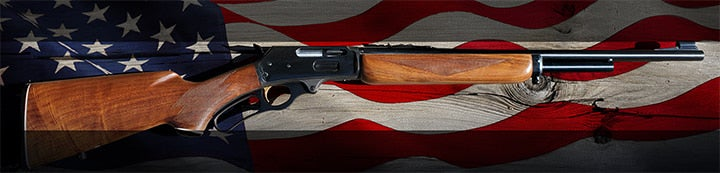1 - 3 of 3 Posts

#### redshirt

·
##### Registered
Joined
·
244 Posts
Discussion Starter · ·
I found S/N info using the search button(thanks swany)
found nothing to date the early ones
Thanks...mike

·
Joined
·
25,048 Posts

#### swany

·
##### Administrator
Joined
·
23,793 Posts
MARLIN Manufacturing DATE CODE.

(22 caliber bolt action & semi-autos were not serial numbered prior to 1969)
Firearm serial numbers were date coded by a prefix within the s/n, as signified below.
For example a Marlin model 39A with a serial number of N1255X would have been made in 1955
while a 1894 in 44 magnum with a serial number of 2510509X, would have equated to 1975

LEVER ACTIONS
ALL FIREARMS

1945 = C 1957 = R 1968 = AD 1981 = 19 1993 = 7
1946 = D 1958 = S 1970 = 70 1982 = 18 1994 = 6
1947 = E 1959 = T 1971 = 71 1983 = 17 1995 = 5
1948 = F 1960 = U 1972 = 72 1984 = 16 1996 = 4
1949 = G 1961 = V 1973 = 73 & 27 1985 = 15 1997 = 3
1950 = H 1962 = W 1974 = 26 1986 = 14 1998 = 2
1951 = J 1963 = Y 1975 = 25 1987 = 13 1999 = 1
1952 = K 1964 = Z 1976 = 24 1988 = 12 2000 = 0
1953 = L 1965 = AA 1977 = 23 1989 = 11
1954 = M 1966 = AB 1978 = 22 1990 = 10
1955 = N 1967 = AC 1979 = 21 1991 = 9
1956 = P 1968 = AD 1980 = 20 1992 = 8

Hope this one helps further. Swany

1 - 3 of 3 Posts